# Bus vs. train

Bus started from point A 10 minutes before the train started from the same place. The bus went an average speed of 49 km/h, train 77 km/h.
To point B train and bus arrived simultaneously.

Calculate time of train journey, if train and bus travelled the same distance.

Result

t =  17.5 min

#### Solution:

49 (t+10) = 77 t

28t = 490

t = 352 = 17.5

Calculated by our simple equation calculator.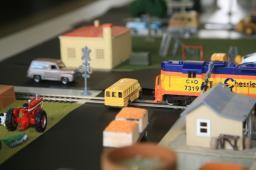Our examples were largely sent or created by pupils and students themselves. Therefore, we would be pleased if you could send us any errors you found, spelling mistakes, or rephasing the example. Thank you!

Leave us a comment of this math problem and its solution (i.e. if it is still somewhat unclear...):Be the first to comment!Tips to related online calculators
Do you have a linear equation or system of equations and looking for its solution? Or do you have quadratic equation?
Do you want to convert length units?
Do you want to convert velocity (speed) units?
Do you want to convert time units like minutes to seconds?

## Next similar math problems:Calculate the radius of the circle whose length is 107 cm larger than its diameter
2. Number of songs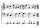Write an expression for the number of songs they need for this show. Evan and Peter have a radio show that has 2 parts. They need 4 fewer than 11 songs in the first part. In the second part, they need 5 fewer than 3 times the number of songs in the first p
3. Pupil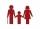I'm a primary school pupil. I attended the exercises of parents with children 1/4 of my age, 1/3 for drawing, and 1/6 for flute. For the first three years of my life, I had no ring, and I never went to two rings at the same time. How old am I?
4. Equal temperature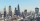The temperatures of the two cities were measured at the same time. The temperature in city A was 60 degrees And rose at a constant rate of 2 degrees per hour. The temperature in city B was 40° and rose at a constant rate of 10° per hour Enter the tim
5. The tourist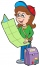The tourist has walked 3/4 of the route. The destination is 12.5 km away. How many kilometers did the route measure?
6. Father and sons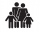Father is 27 and his sons 2 and one year. In how many years will his sons sum up to half his age?
7. Simple equation 1035= 7*3*x what is x?
8. 45th birthdayThis year Mrs. Clever celebrated her 45th birthday. Her three children are now 7.11 and 15 years old. In how many years will Mrs. Clever's age be equal to the sum of her children's years?The fruit basket is five times heavier than the empty basket. Filled is 20kg heavier than empty. Determine the weight of the fruit in the basket.
10. Dividing moneyVilem, Cenek, and Edita divided the money they earned by spreading the leaflet. Vilem got 240 CZK more than Cenek and twice more than Edita. Edita got 400kc less than Vilem.
11. Simple equation 9Solve the following equation: -8y+5=-9y+9
12. Mother and daughterMother is 44 years old, her daughter 14. How many years ago was her mother four times older than her daughter?
13. Simple equation 8Solve the following equation: 36=-(1+7x)-6(-7-x)
14. Brother and sisterWhen I was 8, my sister was 4, now I am 18, how old is my sister?
15. Missing numberBlank +1/6 =3/2 find the missing number
16. Examination 2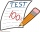In an examination, a teacher awards 2 marks for every correct answer and subtract a mark for every wrong answer. If there are 20 questions and a child scored 25 marks how many correct answer did the child get?
17. Six te 2If 3t-7=5t, then 6t=